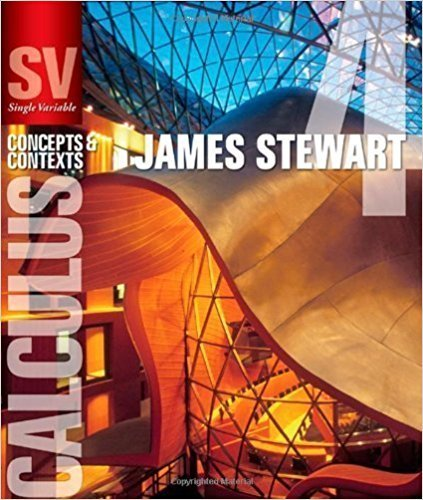×
×

# Solutions for Chapter 1.5: EXPONENTIAL FUNCTIONS## Full solutions for Single Variable Calculus: Concepts and Contexts (Stewart's Calculus Series) | 4th Edition

ISBN: 9780495559726Solutions for Chapter 1.5: EXPONENTIAL FUNCTIONS

Solutions for Chapter 1.5
4 5 0 401 Reviews
15
5
##### ISBN: 9780495559726

Chapter 1.5: EXPONENTIAL FUNCTIONS includes 36 full step-by-step solutions. This textbook survival guide was created for the textbook: Single Variable Calculus: Concepts and Contexts (Stewart's Calculus Series), edition: 4. This expansive textbook survival guide covers the following chapters and their solutions. Since 36 problems in chapter 1.5: EXPONENTIAL FUNCTIONS have been answered, more than 22378 students have viewed full step-by-step solutions from this chapter. Single Variable Calculus: Concepts and Contexts (Stewart's Calculus Series) was written by and is associated to the ISBN: 9780495559726.

Key Calculus Terms and definitions covered in this textbook
• Complex fraction

See Compound fraction.

• Complex number

An expression a + bi, where a (the real part) and b (the imaginary part) are real numbers

• Conversion factor

A ratio equal to 1, used for unit conversion

• Linear correlation

A scatter plot with points clustered along a line. Correlation is positive if the slope is positive and negative if the slope is negative

• Mathematical induction

A process for proving that a statement is true for all natural numbers n by showing that it is true for n = 1 (the anchor) and that, if it is true for n = k, then it must be true for n = k + 1 (the inductive step)

• Negative association

A relationship between two variables in which higher values of one variable are generally associated with lower values of the other variable.

• Reduced row echelon form

A matrix in row echelon form with every column that has a leading 1 having 0’s in all other positions.

• Secant

The function y = sec x.

• Solve graphically

Use a graphical method, including use of a hand sketch or use of a grapher. When appropriate, the approximate solution should be confirmed algebraically

• Standard form of a polar equation of a conic

r = ke 1 e cos ? or r = ke 1 e sin ? ,

• Standard position (angle)

An angle positioned on a rectangular coordinate system with its vertex at the origin and its initial side on the positive x-axis

• Statute mile

5280 feet.

• Stemplot (or stem-and-leaf plot)

An arrangement of a numerical data set into a specific tabular format.

• Tree diagram

A visualization of the Multiplication Principle of Probability.

• Variable

A letter that represents an unspecified number.

• Vector

An ordered pair <a, b> of real numbers in the plane, or an ordered triple <a, b, c> of real numbers in space. A vector has both magnitude and direction.

• Window dimensions

The restrictions on x and y that specify a viewing window. See Viewing window.

• Ymin

The y-value of the bottom of the viewing window.

• yz-plane

The points (0, y, z) in Cartesian space.

• Zero factor property

If ab = 0 , then either a = 0 or b = 0.

×